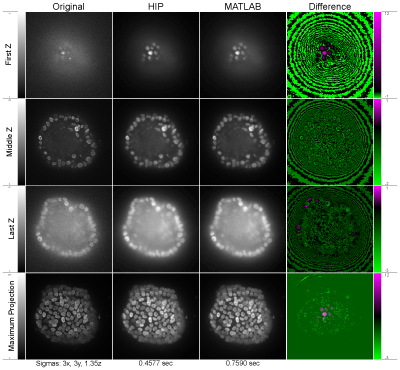### Kernal Re-Normalization

##### Exact Methods for Image Boundaries Eliminating Processing Artifacts

Edge handling in 2-D image processing is often an afterthought, because only pixels very near the edge of the image are affected. However, modern algorithms often utilize very large filters for denoising, creating very wide edge artifacts. Additionally, poor edge handling can significantly affect visualization and interpretation in 3-D volumetric processing.

The three most common approaches for handling edges in image processing software are: zero-padding, last-value padding, and mirroring. Zero-padding adds zeros past the border of the image out to the required filter support. Last-padding repeats the value at the edge of the image past the boundary. Finally, mirroring reverses (mirrors) and replicates the values within the filter neighborhood of the image edge. The simplest approach to edge handling is to zero-pad the image. It is clear, however that for any smoothing or averaging filter this will reduce the image intensity near the edges. This approach will also generally create high-intensity regions on the edges for high-pass or variance filters. The other approaches, such as last-value padding or mirroring, produce much fewer artifacts, but in general still change the total “energy” in the image.

Our kernel renormalization approach begins with the presumption that denoising and smoothing filters should not change the total energy (intensity) of the image, i.e. we assume unit-gain denoising filters. For example, in the case of averaging near an image boundary, this implies that we should simple estimate the average using fewer pixels (ignoring the non-existent pixels past the edge). This idea can be extended to all linear filters by renormalizing the filter so that the filter gain to remain unitary near the edge. The same approach can also be used for non-linear statistical filters, e.g. windowed median, standard-deviation and variance filters, by applying the statistical operation on a truncated window. Qualitatively, this approach provides very good results near boundaries, that also adhere more closely to user expectations of the processing near image boundaries.

##### Image processing using a zero padding scheme

A is a simulated image that has been padded with zeros for a 5x5 neighborhood support. B is a 1-D sample of the image represented by the green box in A. C is the same sample without the padding. The left side of D is a mean filter using B. The right side of D is a mean filter with the kernel re-normalized. E is like D but the kernel is now a Gaussian filter. The resulting values in D and E have been rounded to the next integer value as would be done with integer valued data.##### Image processing using a last value copying scheme

A is a simulated image that has been padded with the last value for a 5x5 neighborhood support. B is a 1-D sample of the image represented by the green box in A. C is the same sample without the padding. The left side of D is a mean filter using B. The right side of D is a mean filter with the kernel re-normalized. E is like D but the kernel is now a Gaussian filter. The resulting values in D and E have been rounded to the next integer value as would be done with integer valued data.

##### Image processing using a mirroring scheme

A is a simulated image that has been padded with a mirror image for a 5x5 neighborhood support. B is a 1-D sample of the image represented by the green box in A. C is the same sample without the padding. The left side of D is a mean filter using B. The right side of D is a mean filter with the kernel re-normalized. E is like D but the kernel is now a Gaussian filter. The resulting values in D and E have been rounded to the next integer value as would be done with integer valued data.##### A comparison of Gaussian smoothing performed on an image with single point precisionThe left most column contains a raw image of cells florescent marked in a three dimensional space. The second column from the left is the resulting image after being smoothed with a large Gaussian kernel using HIP. The Gaussian kernel is smaller in the z direction due to the anisotropic voxel dimensions of the image. The second column from the right was smoothed using a MATLAB Gaussian filter of the same size. The right most column is the difference between the HIP result and the MATLAB result. Positive values are in magenta and negative values are in green. Zero values are in black. All images have been normalized for display with actual ranges listed on the sides. The two middle columns look almost identical, however, the rightmost column shows the structured difference between the two. Note that MATLAB looses energy at image edges with low intensity (first z slice) and gains energy where the intensities are higher (last z slice). Also note the difference in execution time between HIP and MATLAB.​

##### A comparison of Gaussian smoothing performed on an image with unsigned 8 bit integer valuesThe layout is similar to that of figure with single precision. In these integer valued images, one can start to see that the MATLAB version is losing the asymmetry of the underlying data. These differences can significantly change downstream analysis.